## Babies born after 40 weeks gestation have a mean length of 52.2 centimeters (about 20.6 inches). Babies born one month early have a mean len

Question

Babies born after 40 weeks gestation have a mean length of 52.2 centimeters (about 20.6 inches). Babies born one month early have a mean length of 47.4 cm. Assume both standard deviations are 2.5 cm. and the distributions are unimodal and symmetric. a. Find the standardized score (z-score), relative to all U.S. births, for a baby with a birth length of 45 cm. b. Find the standardized score of a birth length of 45 cm. for babies born one month early, using 47.4 as the mean. c. For which group is a birth length of 45 cm more common

in progress 0
3 months 2021-08-21T23:40:14+00:00 1 Answers 0 views 0

a)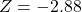b)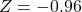c) 40 weeks gestation babies

Step-by-step explanation:

Normal Probability Distribution:

Problems of normal distributions can be solved using the z-score formula.

In a set with mean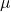and standard deviation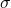, the z-score of a measure X is given by: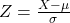The Z-score measures how many standard deviations the measure is from the mean. After finding the Z-score, we look at the z-score table and find the p-value associated with this z-score. This p-value is the probability that the value of the measure is smaller than X, that is, the percentile of X. Subtracting 1 by the p-value, we get the probability that the value of the measure is greater than X.

a. Find the standardized score (z-score), relative to all U.S. births, for a baby with a birth length of 45 cm.

Here, we use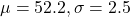. So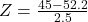b. Find the standardized score of a birth length of 45 cm. for babies born one month early, using 47.4 as the mean.

Here, we use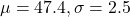. So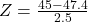c. For which group is a birth length of 45 cm more common?

For each group, the probability is 1 subtracted by the pvalue of Z.

Z = -2.88 has a lower pvalue than Z = -0.96, so for Z = -2.88 the probability 1 – pvalue of Z will be greater. This means that for 40 weeks gestation babies a birth length of 45 cm is more common.